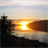# How to link Table value in expression field in Modelbuiler

2261
0
07-02-2014 02:23 AMby
New Contributor
Hi all
I have a tough question about how value can be defined as a variable in expression (the function that I need to use is "Select by attribute").

To be specific, I have two-steps modelbuilder(which means I have to open the modelbuilder two times and then can get the result), the first step aims to calculate mean value and then use this mean value as a threshold value in the second step. Now, I want to make these two steps work together (as a one-step model). This is , I would like to combine this two modelbuilder so that I dont need to input this mean value every time. The ideal situation in my case would be like this: model can automatically calculated mean value and input as a threshold for second processing. In this case, I have to define this mean value as a variable, as this mean value is not constant but variable(Because I have too many works). Before this one-step model has been created, I have to type mean value manually as a input for the second step. For example, I obtained the mean value from the first step, for instance, 500, and then I make a expression : Features ?? 500. then all the features that are less than 500 would be selected.

If I make this one-step modelbuilder possible, I could define this mean value as a variable , then I could probably make a query in expression, such as feature ?? value.mean? In this case, I dont need to know exactly what the mean value is, instead, I just use a SQL language and ArcGIS can automatically input every mean value calculated by first steps as a threshold for the second step.

Note: I actually have two files in the second processing, one is this mean value table, another is one shpfile layer. I want to use this mean value as a threshold to acquire those required features from the shpfile layer. However, I dont have any background on SQL in ArcGIS and I just wonder if anyone could help me. I would be much appreciate.

Please note the table calculated by the first steps was stored in one row, which means not all the value stored in table need to be used. Below is a format of table calculated by the first step. I only need that mean value.
[ATTACH=CONFIG]35034[/ATTACH]
Tags (3)
0 Replies